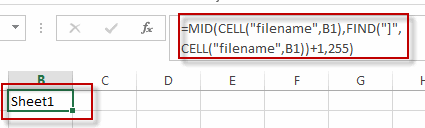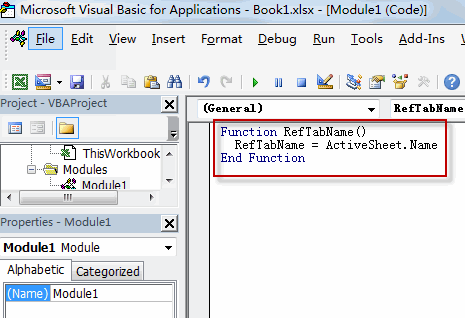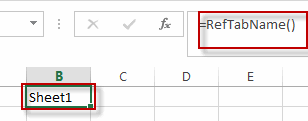# Reference Tab Name

This post will guide you how to reference a tab name in one cell in Excel. How do I reference a tab name in cell with a formula in Excel. Can anyone tell me the formula on displaying the name of the current worksheet name in a cell on the Sheet.

## Reference Tab Name with a Formula

To reference a tab name in a cell with a formula in Excel, you can create a formula based on the MID function, the Cell function, and the FIND function.

Assuming that you want to reference the current worksheet name in cell B1, you can use the following formula to achieve it. Like this:

`=MID(CELL("filename",B1),FIND("]",CELL("filename",B1))+1,255)`

Type this formula into the formula box of cell B1, and press Enter key to apply this formula.You will see that the tab name of the current worksheet has been displayed into the cell B1.

## Reference Tab Name with a User Defined Function

If you want to reference the sheet tab name quickly, you can write a User defined function in Excel VBA. Just do the following steps:

#1 open your excel workbook and then click on “Visual Basic” command under DEVELOPER Tab, or just press “ALT+F11” shortcut.#2 then the “Visual Basic Editor” window will appear.

#3 click “Insert” ->”Module” to create a new module.#4 paste the below VBA code into the code window. Then clicking “Save” button.```Function RefTabName()

RefTabName = ActiveSheet.Name

End Function```

#5 back to the current worksheet, try to enter the below formula in Cell B1.

`=RefTabName()`You will see the sheet tab name is displayed in cell B1.

### Related Functions

• Excel FIND function
The Excel FIND function returns the position of the first text string (sub string) within another text string.The syntax of the FIND function is as below:= FIND(find_text, within_text,[start_num])…
• Excel MID function
The Excel MID function returns a substring from a text string at the position that you specify.The syntax of the MID function is as below:= MID (text, start_num, num_chars)….
• Excel CELL function
The Excel CELL function returns information about the formatting, location, size, or contents of a cell.The syntax of the CELL function is as below:= CELL (info_type,[reference])…